# Completely-integrable differential equation

(diff) ← Older revision | Latest revision (diff) | Newer revision → (diff)

An equation of the form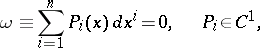(*)

for which an-dimensional integral manifold passes through each point of a certain domain in the space. A necessary and sufficient condition for complete integrability of the differential equation (*) is the Frobenius condition, whereis the symbol of the exterior product . If, this condition has the form: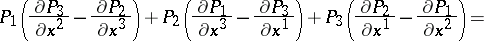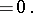Instead of equation (*) the following system of equations is sometimes considered :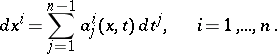In this case the conditions of complete integrability assume the form: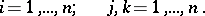The family of integral manifolds of a completely-integrable differential equation is a foliation .

How to Cite This Entry:
Completely-integrable differential equation. Encyclopedia of Mathematics. URL: http://encyclopediaofmath.org/index.php?title=Completely-integrable_differential_equation&oldid=13469
This article was adapted from an original article by L.E. Reizin' (originator), which appeared in Encyclopedia of Mathematics - ISBN 1402006098. See original article